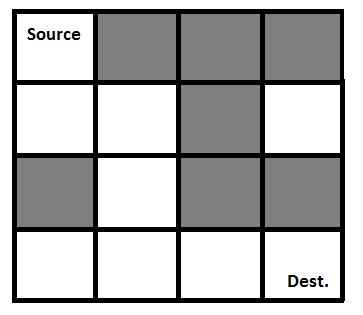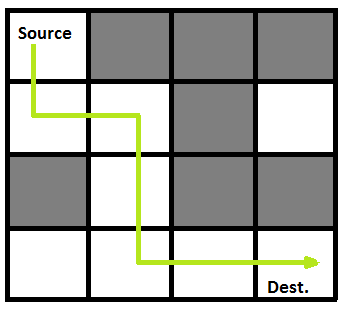# Rat inside a maze (Using Backtracking Algorithm)

Consider a situation where a rat is dropped at the source and the rat has to reach the destination node in the best path possible. The black nodes in the figure mean to block the way for the rat and hence rat has to reach destination following the path in white nodes. Write a program such that the rat reaches the destination with the best possible route in the given maze.

Solution:

Step 1: Convert the given maze into a readable matrix where the black nodes are represented by zero's and white nodes are represented by one. Now a program has to be written to traverse on the ones to reach the destination node.

This problem can typically be solved by two methods.

One is by the native method of finding out all possible paths and then finding the minimum distance or minimum hops path that needs to be taken by the rat to reach the destination. This procedure is complex in nature and a lot of memory is being used to store all possible paths and later check the best possible route in it.

Instead of the above method, we could use backtracking algorithm:

Backtracking algorithm can be defined as an algorithm used to find all possible solutions in a problem. This algorithm traverses one node at a time and incrementally builds the solutions. In each step, if the algorithm recognises any block that might not be used to find the best path that block is cancelled, i.e in this case that block is also marked as '0'. This reduces the number of trails and avoids the system from storing all possible path traversals till the last step of the program.

Following is an example maze.

` Gray blocks are dead ends (value = 0). `Binary representation of the matrix is given below:

```                {1, 0, 0, 0}
{1, 1, 0, 1}
{0, 1, 0, 0}
{1, 1, 1, 1}
```

The best path solution is marked.solution matrix /output of program for the above input matrix.

```                {1, 0, 0, 0}
{1, 1, 0, 0}
{0, 1, 0, 0}
{0, 1, 1, 1}
All enteries in solution path are marked as 1.```

## C/C++ Implementation of Backtracking solution

```/* C/C++ program to solve Rat in a Maze problem using
backtracking */
#include<stdio.h>

// Maze size
#define N 4

bool solveMazeUtil(int maze[N][N], int x, int y, int sol[N][N]);

/* A utility function to print solution matrix sol[N][N] */
void printSolution(int sol[N][N])
{
for (int i = 0; i < N; i++)
{
for (int j = 0; j < N; j++)
printf(" %d ", sol[i][j]);
printf("\n");
}
}

/* A utility function to check if x,y is valid index for N*N maze */
bool isSafe(int maze[N][N], int x, int y)
{
// if (x,y outside maze) return false
if(x >= 0 && x < N && y >= 0 && y < N && maze[x][y] == 1)
return true;

return false;
}

/* This function solves the Maze problem using Backtracking.  It mainly
uses solveMazeUtil() to solve the problem. It returns false if no
path is possible, otherwise return true and prints the path in the
form of 1s. Please note that there may be more than one solutions,
this function prints one of the feasible solutions.*/
bool solveMaze(int maze[N][N])
{
int sol[N][N] = { {0, 0, 0, 0},
{0, 0, 0, 0},
{0, 0, 0, 0},
{0, 0, 0, 0}
};

if(solveMazeUtil(maze, 0, 0, sol) == false)
{
printf("Solution doesn't exist");
return false;
}

printSolution(sol);
return true;
}

/* A recursive utility function to solve Maze problem */
bool solveMazeUtil(int maze[N][N], int x, int y, int sol[N][N])
{
// if (x,y is goal) return true
if(x == N-1 && y == N-1)
{
sol[x][y] = 1;
return true;
}

// Check if maze[x][y] is valid
if(isSafe(maze, x, y) == true)
{
// mark x,y as part of solution path
sol[x][y] = 1;

/* Move forward in x direction */
if (solveMazeUtil(maze, x+1, y, sol) == true)
return true;

/* If moving in x direction doesn't give solution then
Move down in y direction  */
if (solveMazeUtil(maze, x, y+1, sol) == true)
return true;

/* If none of the above movements work then BACKTRACK:
unmark x,y as part of solution path */
sol[x][y] = 0;
return false;
}

return false;
}

// driver program to test above function
int main()
{
int maze[N][N]  =  { {1, 0, 0, 0},
{1, 1, 0, 1},
{0, 1, 0, 0},
{1, 1, 1, 1}
};

solveMaze(maze);
return 0;
}
```

## Java Implementation of Backtracking solution

```/* Java program to solve Rat in a Maze problem using
backtracking */

public class RatMaze
{
final int N = 4;

/* A utility function to print solution matrix
sol[N][N] */
void printSolution(int sol[][])
{
for (int i = 0; i < N; i++)
{
for (int j = 0; j < N; j++)
System.out.print(" " + sol[i][j] +
" ");
System.out.println();
}
}

/* A utility function to check if x,y is valid
index for N*N maze */
boolean isSafe(int maze[][], int x, int y)
{
// if (x,y outside maze) return false
return (x >= 0 && x < N && y >= 0 &&
y < N && maze[x][y] == 1);
}

/* This function solves the Maze problem using
Backtracking. It mainly uses solveMazeUtil()
to solve the problem. It returns false if no
path is possible, otherwise return true and
prints the path in the form of 1s. Please note
that there may be more than one solutions, this
function prints one of the feasible solutions.*/
boolean solveMaze(int maze[][])
{
int sol[][] = {{0, 0, 0, 0},
{0, 0, 0, 0},
{0, 0, 0, 0},
{0, 0, 0, 0}
};

if (solveMazeUtil(maze, 0, 0, sol) == false)
{
System.out.print("Solution doesn't exist");
return false;
}

printSolution(sol);
return true;
}

/* A recursive utility function to solve Maze
problem */
boolean solveMazeUtil(int maze[][], int x, int y,
int sol[][])
{
// if (x,y is goal) return true
if (x == N - 1 && y == N - 1)
{
sol[x][y] = 1;
return true;
}

// Check if maze[x][y] is valid
if (isSafe(maze, x, y) == true)
{
// mark x,y as part of solution path
sol[x][y] = 1;

/* Move forward in x direction */
if (solveMazeUtil(maze, x + 1, y, sol))
return true;

/* If moving in x direction doesn't give
solution then  Move down in y direction */
if (solveMazeUtil(maze, x, y + 1, sol))
return true;

/* If none of the above movements work then
BACKTRACK: unmark x,y as part of solution
path */
sol[x][y] = 0;
return false;
}

return false;
}

public static void main(String args[])
{
RatMaze rat = new RatMaze();
int maze[][] = {{1, 0, 0, 0},
{1, 1, 0, 1},
{0, 1, 0, 0},
{1, 1, 1, 1}
};
rat.solveMaze(maze);
}
}

```

## Python3 Implementation of Backtracking solution

```# Python3 program to solve Rat in a Maze
# problem using backracking

# Maze size
N = 4

# A utility function to print solution matrix sol
def printSolution( sol ):

for i in sol:
for j in i:
print(str(j) + " ", end="")
print("")

# A utility function to check if x,y is valid
# index for N*N Maze
def isSafe( maze, x, y ):

if x >= 0 and x < N and y >= 0 and y < N and maze[x][y] == 1:
return True

return False

""" This function solves the Maze problem using Backtracking.
It mainly uses solveMazeUtil() to solve the problem. It
returns false if no path is possible, otherwise return
true and prints the path in the form of 1s. Please note
that there may be more than one solutions, this function
prints one of the feasable solutions. """
def solveMaze( maze ):

# Creating a 4 * 4 2-D list
sol = [ [ 0 for j in range(4) ] for i in range(4) ]

if solveMazeUtil(maze, 0, 0, sol) == False:
print("Solution doesn't exist");
return False

printSolution(sol)
return True

# A recursive utility function to solve Maze problem
def solveMazeUtil(maze, x, y, sol):

#if (x,y is goal) return True
if x == N - 1 and y == N - 1:
sol[x][y] = 1
return True

# Check if maze[x][y] is valid
if isSafe(maze, x, y) == True:
# mark x, y as part of solution path
sol[x][y] = 1

# Move forward in x direction
if solveMazeUtil(maze, x + 1, y, sol) == True:
return True

# If moving in x direction doesn't give solution
# then Move down in y direction
if solveMazeUtil(maze, x, y + 1, sol) == True:
return True

# If none of the above movements work then
# BACKTRACK: unmark x,y as part of solution path
sol[x][y] = 0
return False

# Driver program to test above function
if __name__ == "__main__":
# Initialising the maze
maze = [ [1, 0, 0, 0],
[1, 1, 0, 1],
[0, 1, 0, 0],
[1, 1, 1, 1] ]

solveMaze(maze)

```

Output: The 1 values show the path for rat

``` 1  0  0  0
1  1  0  0
0  1  0  0
0  1  1  1 ```

POST A NEW COMMENT

• Input (stdin)

Output (stdout)

Input (stdin)

Expected Output

Compiler Message

Input (stdin)

`2    3`

`5`
`5`
`5`